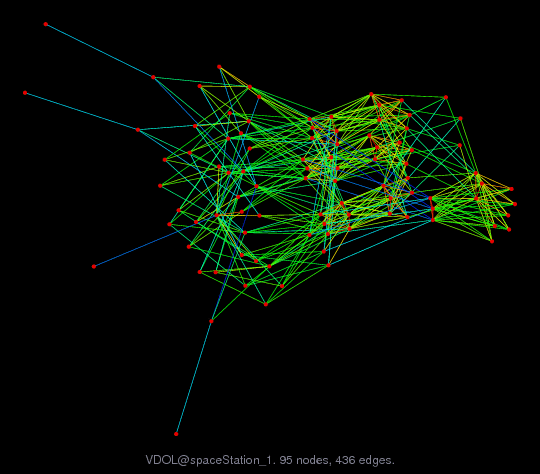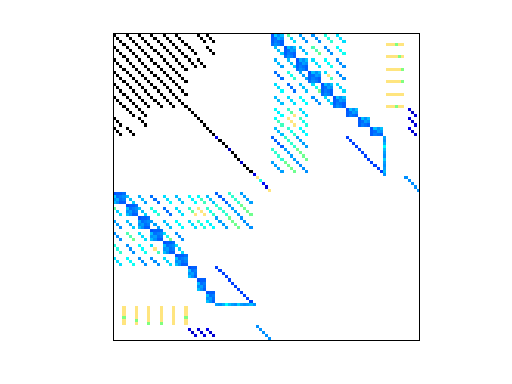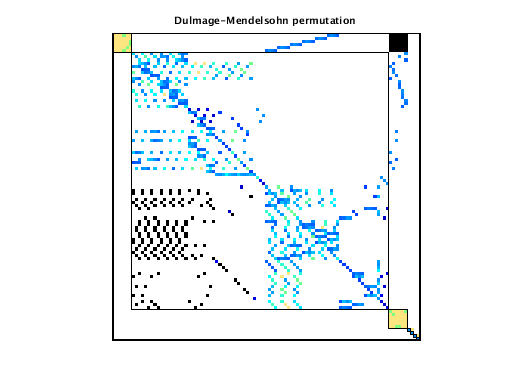Matrix: VDOL/spaceStation_1

Description: spaceStation optimal control problem (matrix 1 of 14)(undirected graph drawing)• Matrix group: VDOL
• download as a MATLAB mat-file, file size: 41 KB. Use UFget(2734) or UFget('VDOL/spaceStation_1') in MATLAB.

 Matrix properties number of rows 99 number of columns 99 nonzeros 927 structural full rank? yes structural rank 99 # of blocks from dmperm 7 # strongly connected comp. 3 explicit zero entries 0 nonzero pattern symmetry symmetric numeric value symmetry symmetric type real structure symmetric Cholesky candidate? no positive definite? no

 author B. Senses, A. Rao editor T. Davis date 2015 kind optimal control problem 2D/3D problem? no

 Additional fields size and type b full 99-by-1 rowname full 99-by-97 mapping full 99-by-1

Notes:

```Optimal control problem, Vehicle Dynamics & Optimization Lab, UF
Anil Rao and Begum Senses, University of Florida
http://vdol.mae.ufl.edu

This matrix arises from an optimal control problem described below.
Each optimal control problem gives rise to a sequence of matrices of
different sizes when they are being solved inside GPOPS, an optimal
control solver created by Anil Rao, Begum Senses, and others at in VDOL
lab at the University of Florida.  This is one of the matrices in one
of these problems.  The matrix is symmetric indefinite.

Rao, Senses, and Davis have created a graph coarsening strategy
that matches pairs of nodes.  The mapping is given for this matrix,
where map(i)=k means that node i in the original graph is mapped to
node k in the smaller graph.  map(i)=map(j)=k means that both nodes
i and j are mapped to the same node k, and thus nodes i and j have
been merged.

This matrix consists of a set of nodes (rows/columns) and the
names of these rows/cols are given

Anil Rao, Begum Sense, and Tim Davis, 2015.

VDOL/spaceStation

Space station attitude optimal control problem is taken from
Ref.~\cite{betts2010practical}. The goal of the space station
attitude control problem is to determine the state and the control
that minimize the magnitude of the final momentum while the space
statition reaches an orientation at the final time that can be
maintained without utilizing additional control torque. The state of
the system is defined by the angular velocity of the spacecraft with
respect to an inertial reference frame, Euler-Rodriguez parameters
used to defined the vehicle attitude, and the angular momentum of the
control moment gyroscope and the control of the system is the torque.
The specified accuracy tolerance of \$10^{-7}\$ were satisfied after
thirteen mesh iterations. As the mesh refinement proceeds, the size
of the KKT matrices increases from 99 to 1640.
```

 Ordering statistics: result nnz(chol(P*(A+A'+s*I)*P')) with AMD 1,188 Cholesky flop count 2.3e+04 nnz(L+U), no partial pivoting, with AMD 2,277 nnz(V) for QR, upper bound nnz(L) for LU, with COLAMD 1,773 nnz(R) for QR, upper bound nnz(U) for LU, with COLAMD 2,922